• 目录前言分支定界算法列生成算法步骤参考文献 前言 简述分支定价算法原理，如有错误可私信，谢谢。部分截图来自本人汇报所用PPT以及参考文献。 分支定界算法 分支定价算法(branch and price, B&P）=分支定界...
目录前言分支定界算法列生成算法步骤参考文献
前言
简述分支定价算法原理，如有错误可私信，谢谢。部分截图来自本人汇报所用PPT以及参考文献。以下均以最小化问题为例。
分支定界算法
分支定价算法(branch and price, B&P）=分支定界(branch and bound, B&B）+列生成(column generation, CG, 线性规划)。其中列生成算法用于求节点的下界，即节点松弛模型的最优解。列生成算法因其求解方法的本质会大大减少计算量，求解的并非节点松弛模型本身，而是受限制的松弛模型，即减少了决策变量规模。分支定价算法其他步骤与分支定界算法相同，只是下界计算由列生成算法完成。如下为流程图。列生成算法列生成算法所求解的问题大都决策变量规模非常大，相应地其约束系数也非常多（如上图每个xj对应一组{a1j,…,aij,…,anj}），不可能同时表达出来一起求解，典型问题就是切卷纸的问题，顾客需要不同长度的卷纸若干，需要对固定长度的卷纸进行裁剪。对卷纸而言可行的裁剪方案的约束条件是不超过卷纸的固定长度（可能会有巨多种），对整个问题而言，就是找到一个解决方案（方案由裁剪方案与裁剪数量组成，如裁剪方案1裁剪5卷纸，裁剪方案2裁剪3卷纸可得到最终结果）得到顾客想要的若干不同长度卷纸，并使用最少的原固定长度的卷纸。列生成特点为无需列出所有可行的裁剪方案(恢复成多)再进行求解，只需先列出几个裁剪方案然后随着求解这几个裁剪方案下的原问题（主问题，Master Problem）的受限问题（Restricted Master Problem），再不断迭代增加对问题有益的裁剪方案最终求出松弛问题的最优解。
步骤
（如上图）
1、根据原问题，选取几个可行列（可行裁剪方案），得到受限问题模型
2、通过受限问题RMP获得检验数以构建子问题的方程，子问题的约束条件即为可行列的生成条件（裁剪方案需要满足卷纸固定长度的限制，例如5米长的卷纸，只能裁剪成3米1个，2米1个；不能是4米1个，2米1个）
子问题表达：子问题目标函数中检验数pi来自于RMP问题，通过RMP的基向量和非基向量划分后的对应参数c_B和B计算得到（参考单纯形法计算过程，参考）。需要将RMP问题标准化得到基变量非基变量，将其对应参数计算检验数，并用以求解子问题，得到新的可行列加入RMP问题。3、求解子问题，子问题的解若对应目标函数（检验数）小于0，那么将解y（可行列）加入受限问题，循环步骤2~3，否则若子问题的解对应目标值不小于0，则循环结束
4、求当前受限问题RMP的最优解，即分支定价中该节点的下界
注：建议配合给出的参考中的链接一起理解，步骤2~3的依据是单纯形法中进基出基的判断方法（进基出基后可得到子问题目标值中的检验数），当基确定后（通过每列的检验数判断），RMP问题最优解则可得出，也就是分支定价过程中该节点的下界。
参考文献
 列生成：https://www.cnblogs.com/dengfaheng/p/11249879.html
https://www.jianshu.com/p/8be0083886ed
https://www.cnblogs.com/cruelty_angel/p/10493527.html
 分支定界：https://www.cnblogs.com/dengfaheng/p/11225612.html
 分支定价：https://my.oschina.net/u/4354879/blog/3432783
李播.基于分支定价的手术计划调度研究


展开全文• 在上一篇《机器学习1》中提到梯度下降算法出了代数表达式，来看一下代数实现下面我们把它放到python 3里面，转变成代码的形式去实现梯度下降。import numpy as np X=2*np.random.rand(100,1)#生成训练函数(特征...
在上一篇《机器学习1》中提到梯度下降算法并列出了代数表达式，来看一下代数实现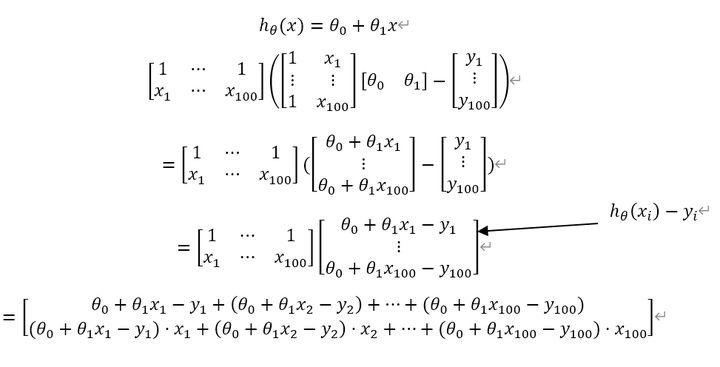下面我们把它放到python 3里面，转变成代码的形式去实现梯度下降。import numpy as np

X=2*np.random.rand(100,1)#生成训练函数(特征部分)
y=4+3*X+np.random.randn(100,1)   #生成训练数据(标签部分)
plt.plot(X,y,'b.')  #画图
plt.xlabel('$x_1$',fontsize=18)
plt.ylabel('$y$',rotation=0,fontsize=18)
plt.axis([0,2,0,15])
save_fig('generated_data_plot')  #保存图片
plt.show()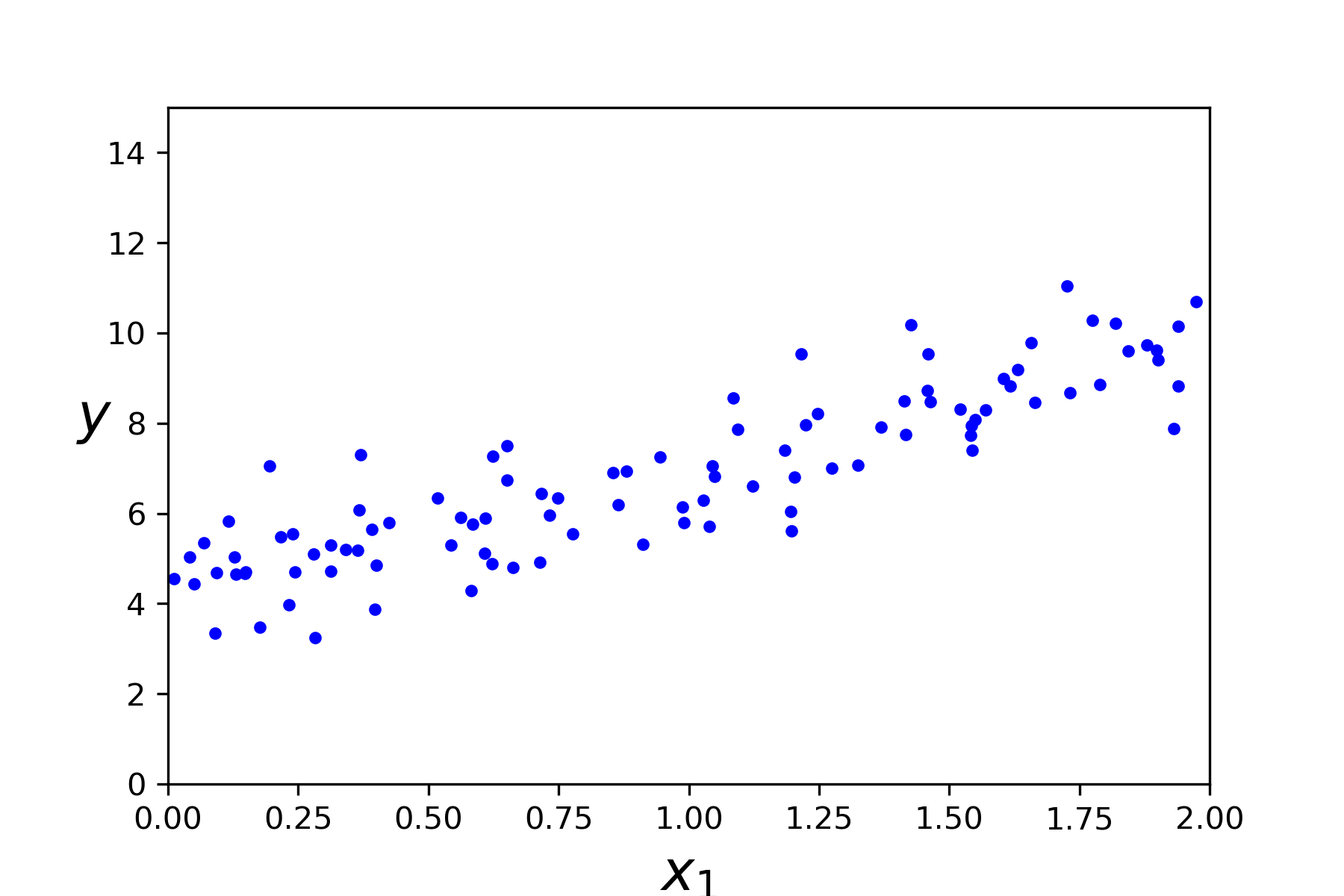1.批量梯度下降算法在上面代码的基础上,我们还要添加新特征,创建测试数据。设置步长，数据集个数，迭代次数def plot_gradient_descent(theta,eta,theta_path=None):
m=len(X_b)
plt.plot(X,y,'b.')
n_iterations=1000     #迭代次数
for iteration in range(n_iterations):
if iteration <10:    #画线
y_predict=X_new_b.dot(theta)
style='b-'
plt.plot(X_new,y_predict,style)
if theta_path is not None:
theta_path.append(theta)
plt.xlabel('$x_1$',fontsize=18)
plt.axis([0,2,0,15])
plt.title(r'$eta={}$'.format(eta),fontsize=16)采用三种步长逼近: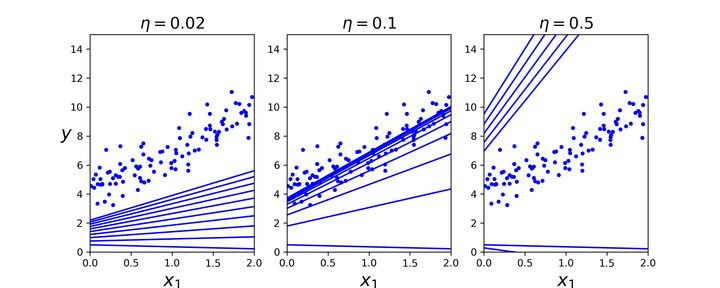2.随机梯度下降算法n_epochs=50

theta=np.random.randn(2,1)     #随机初始化

for epoch in range(n_epochs):
for i in range(m):
if epoch==0 and i<20:
y_predict=X_new_b.dot(theta)
style='b-'
plt.plot(X_new,y_predict,style)
random_index=np.random.randint(m)
xi=X_b[random_index:random_index+1]
yi=y[random_index:random_index+1]
eta=0.1
theta_path_sgd.append(theta)

plt.plot(X,y,'b.')
plt.xlabel('$x_1$',fontsize=18)
plt.ylabel('$y$',rotation=0,fontsize=18)
plt.axis([0,2,0,15])
save_fig('sgd_plot')
plt.show()
theta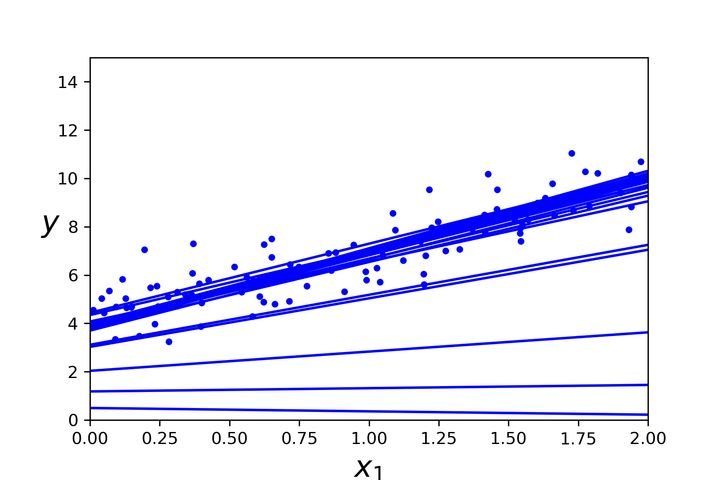3.小批量梯度下降算法theta_path_mgd=[]

n_iterations=50
minibatch_size=20

np.random.seed(42)
theta=np.random.randn(2,1)

for epoch in range(n_iterations):
shuffled_indices=np.random.permutation(m)
X_b_shuffled=X_b[shuffled_indices]
y_shuffled=y[shuffled_indices]
for i in range(0,m,minibatch_size):
xi=X_b_shuffled[i:i+minibatch_size]
yi=y_shuffled[i:i+minibatch_size]
eta=0.1
theta_path_mgd.append(theta)泰勒公式(泰勒展开式)是用一个函数在某点的信息，描述其附近取值的公式。如果函数足够平滑，在已知函数在某一点的各阶导数值的情况下，泰勒公式可以利用这些导数值来做系数，构建一个多项式近似函数，求得在这一点的邻域中的值。所以泰勒公式是做什么用的？简单来讲就是用一个多项式函数去逼近一个给定的函数(即尽量使多项式函数图像拟合给定的函数图像)，注意，逼近的时候一定是从函数图像上的某个点展开。如果一个非常复杂函数，想求其某点的值，直接求无法实现，这时候可以使用泰勒公式去近似的求该值，这是泰勒公式的应用之一。泰勒公式在机器学习中主要应用于梯度迭代。1.一元泰勒展开式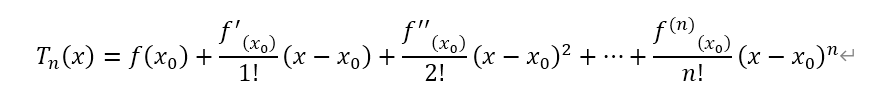2.二元泰勒展开式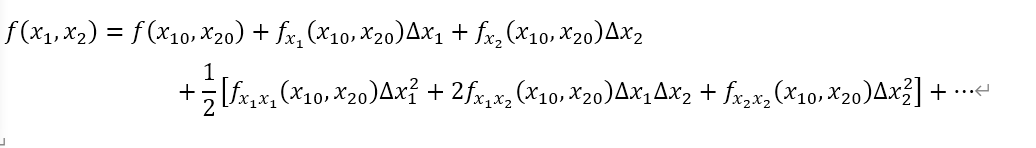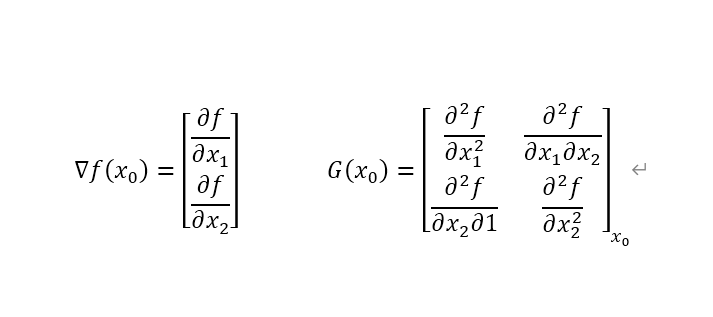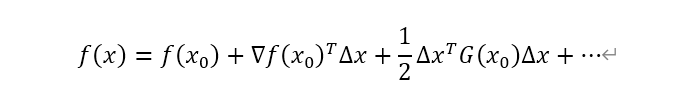展开全文• 电脑自动生成数独游戏的谜题要得出所有满足条件的组合确实不是件容易...所以，算法步骤是：1.往第一行或第一随机填1-9的数字2.调用解数独算法得到一个结果3.每行随机遮掉1-8个数字。如果需要较大的难度，也可以将1-
电脑自动生成数独游戏的谜题
要得出所有满足条件的组合确实不是件容易的事情（主要是很多，打印起来很慢） 。但偶们的目标只是每次能得到一个新的组合，然后从格子里面随机遮掉一些数字就可以了。所以只需要在解数独游戏算法的基础上稍作修改即可。
所以，算法的步骤是：
1.往第一行或第一列随机填1-9的数字
2.调用解数独算法得到一个结果
3.每行随机遮掉1-8个数字。如果需要较大的难度，也可以将1-8改为2-8或3-8，等等。
以下是console工程的代码：
// sudoku.cpp : 定义控制台应用程序的入口点。// by superarhow(superarhow@hotmail.com)
#include "stdafx.h"
#include "conio.h"
/***************** 广告位招租 *********************/
/* 为了代码好看^^ */#define SUCCESS  1#define _FAILED  0
/* 地图类型9*9的char，每个char从0-9，0表示待填 */typedef char MAPTYPE;/*  行数据，同时用作“可能性”数据。如LINETYPE a; 当a为真时表示   当前位置可填1，a为真时表示可填2，以此类推 */typedef char LINETYPE;
typedef void (*ONMAPOKCALLBACK)(MAPTYPE map);
/* 打印地图 */void dump_map(MAPTYPE dest){ for ( int j = 0; j < 9; j++ ) {  for ( int i = 0; i < 9; i++ )  {   printf("%d ", dest[i][j]);  }  printf("/n"); } printf("/n");}
int fill_line(MAPTYPE dest, int line, ONMAPOKCALLBACK callback);
/* 填下一个格子。本行的可能性已在调用前算好，要考虑的是列的可能性和九宫格的可能性 *//* nums_possible : array (0-8) means possible of number (1-9) */int fill_pos(MAPTYPE dest, LINETYPE nums_possible, int line, int pos, ONMAPOKCALLBACK callback){ if ( pos >= 9 ) {  return fill_line(dest, line + 1, callback); } if ( dest[pos][line] != 0 ) return fill_pos(dest, nums_possible, line, pos + 1, callback); for ( int i = 0; i < 9; i++ ) {  if ( !nums_possible[i] ) continue;  /* 检查本列是否重复 */  int vetical_failed = 0;  for ( int j = 0; j < 9; j++ )    if ( dest[pos][j] == i + 1 )   {    vetical_failed = 1;    break;   }  if ( vetical_failed ) continue;   /* 检查九宫格是否重复 */  int nine_failed = 0;  int m = pos / 3;  int n = line / 3;  m *= 3;  n *= 3;  for ( int y = n; y < n + 3; y++ )  {   for ( int x = m; x < m + 3; x++ )   {    if ( dest[x][y] == i + 1 )     {     nine_failed = 1;     break;    }   }   if ( nine_failed ) break;  }  if ( nine_failed ) continue;  /* all ok, try next position */  dest[pos][line] = i + 1;  nums_possible[i] = 0;  if ( fill_pos(dest, nums_possible, line, pos + 1, callback) )   {   /* 本行已全部OK，尝试下一行 */   if ( fill_line(dest, line + 1, callback) ) return SUCCESS;   /* 下一行失败，重新尝试本位置的剩余可能性 */  }  nums_possible[i] = 1;  dest[pos][line] = 0; } return _FAILED;}
/* 填下一行 */int fill_line(MAPTYPE dest, int line, ONMAPOKCALLBACK callback){ if ( line >= 9 ) {  /* copy map */  callback(dest);  return SUCCESS; } LINETYPE nums; LINETYPE saveline; /* calc possibility(for the current line) */ for ( int i = 0; i < 9; i++ ) nums[i] = 1; /* all can be */ for ( int i = 0; i < 9; i++ ) {  char n = dest[i][line];  /* save line */  saveline[i] = dest[i][line];  if ( n != 0 ) nums[n - 1] = 0; /* appears */ } if ( !fill_pos(dest, nums, line, 0, callback) ) {  /* restore line */  for ( int i = 0; i < 9; i++ ) dest[i][line] = saveline[i];  return _FAILED; } return SUCCESS;}
MAPTYPE g_result;
void on_map_ok(MAPTYPE map){ memcpy(g_result, map, sizeof(MAPTYPE));}
#include "windows.h"
int _tmain(int argc, _TCHAR* argv[]){ MAPTYPE dest; memset(dest, 0, sizeof(MAPTYPE)); srand( GetTickCount() ); /* 随机填充第一行 */ char ch = { 1, 2, 3, 4, 5, 6, 7, 8, 9 }; for ( int i = 0; i < 9; i++ ) {  int p = rand() % 9;  char t = ch[p];  ch[p] = ch[i];  ch[i] = t; } for ( int i = 0; i < 9; i++ ) dest[i] = ch[i]; if ( fill_line(dest, 0, on_map_ok) )  {  /* 修剪掉一些块 */  for ( int i = 0; i < 9; i++ )   {   /* 调整n的取值范围可改变难度 %6 + 3是比较难的 */   int n = (rand() % 6) + 3;   for ( int j = 0; j < 9; j++ ) ch[j] = j; /* ch: index to erase */   for ( int j = 0; j < 9; j++ )   {    int p = rand() % 9;    char t = ch[p];    ch[p] = ch[i];    ch[i] = t;   }   for ( int j = 0; j < n; j++ ) g_result[ch[j]][i] = 0;  }  dump_map(g_result); } getch(); return 0;}

展开全文callback
• 重复以上步骤直到列表为空，迷宫生成完毕。这种方式生成的迷宫难度高，岔口多。 效果： 代码： import random import numpy as np from matplotlib import pyplot as plt def build_twist(num_rows, num_cols): # ...
• 最小生成树 1.克鲁斯卡尔算法 ∙\bullet∙克鲁斯卡尔算法的实质就是加边，先对边进行从小到大排序，然后再从小的边开始加进树里，但是不能构成环。重复上述步骤，直至树里面有n-1条边（总共有n个结点） 原始图：...
最小生成树
1.克鲁斯卡尔算法
$\bullet$克鲁斯卡尔算法的实质就是加边，先对边进行从小到大排序，然后再从小的边开始加进树里，但是不能构成环。重复上述步骤，直至树里面有n-1条边（总共有n个结点）
原始图：（从1号点开始）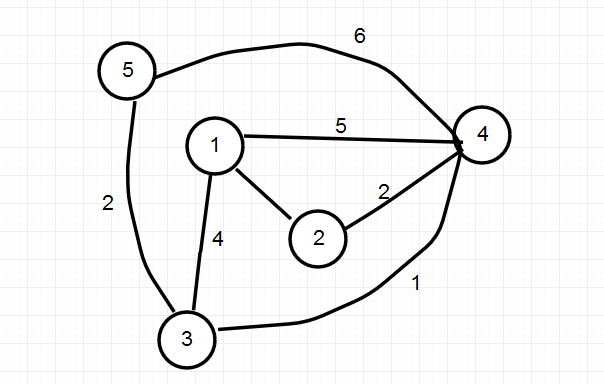第一次：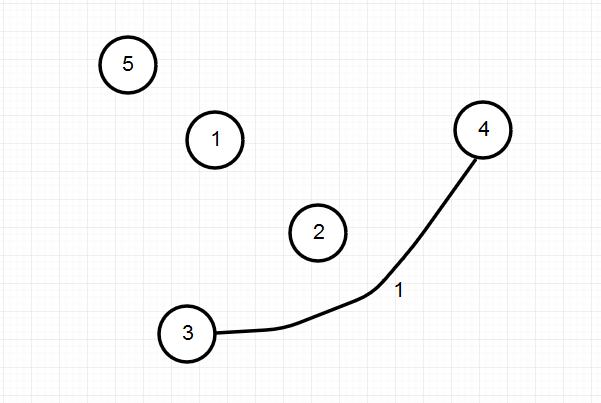第二次: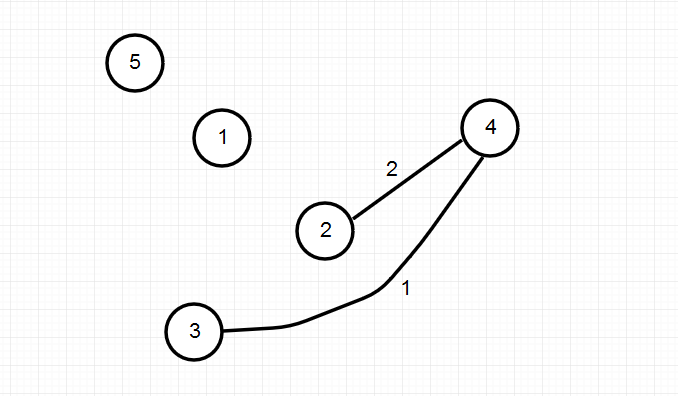第三次：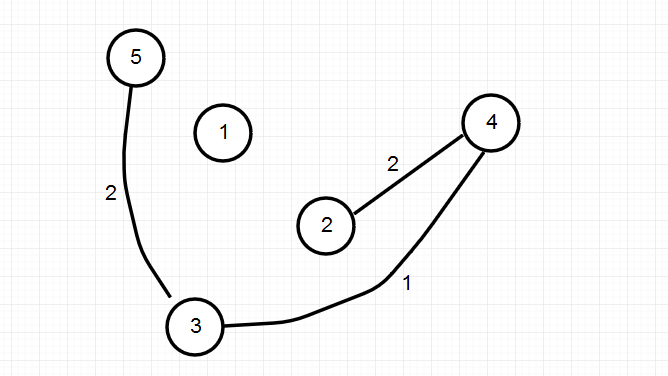第四次：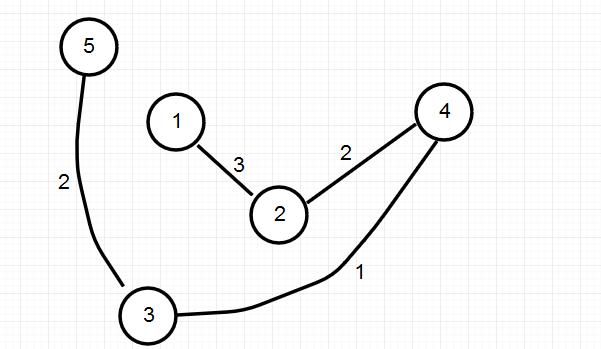好了，最小生成树就构造好了。
$\bullet$例题：
某省调查乡村交通状况，得到的统计表中列出了任意两村庄间的距离。省政府“畅通工程”的目标是使全省任何两个村庄间都可以实现公路交通（但不一定有直接的公路相连，只要能间接通过公路可达即可），并要求铺设的公路总长度为最小。请计算最小的公路总长度。
Input
测试输入包含若干测试用例。每个测试用例的第1行给出村庄数目N ( < 100 )；随后的N(N-1)/2行对应村庄间的距离，每行给出一对正整数，分别是两个村庄的编号，以及此两村庄间的距离。为简单起见，村庄从1到N编号。
当N为0时，输入结束，该用例不被处理。
Output
对每个测试用例，在1行里输出最小的公路总长度。
Sample Input
3
1 2 1
1 3 2
2 3 4
4
1 2 1
1 3 4
1 4 1
2 3 3
2 4 2
3 4 5
0

Sample Output
3
5

$\bullet$代码运用到了并查集：
#include<stdio.h>
#include<string.h>
#include<algorithm>
#include<queue>
#include<vector>
#include<set>
#include<iostream>
#include<queue>
#include<string>
using namespace std;
const int maxn=1e4+10;
const int inf=999999999;
int par[maxn];
int k,m,n;
struct node
{
int s,e,val;
}e[maxn];

int cmp(node a,node b)
{
return a.val<b.val;
}

int Find(int x)
{
if(x==par[x])return x;
else {
par[x]=Find(par[x]);
return  par[x];
}
}

void join(int a,int b)
{
int fa=Find(a);
int fb=Find(b);
if(fa!=fb){
par[fb]=fa;
}
}

void init()
{
for(int i=1;i<=n*(n-1)/2;i++)par[i]=i;
memset(e,0,sizeof(e));
}

int main()
{
while(scanf("%d",&n)&&n)
{
init();
int N=n*(n-1)/2;
for(int i=1;i<=N;i++){
int a,b,c;
scanf("%d%d%d",&a,&b,&c);
e[i]={a,b,c};
}
sort(e+1,e+N+1,cmp);//对边进行排序
int sum=0;
int flag=0;
for(int i=1;i<=N;i++){
if(Find(e[i].s)!=Find(e[i].e)&&(flag<=n-1)){//要合并的两个点的祖先结点不能一样，一样就要构成环了，这是不符合最小生成树的定义的
join(e[i].s,e[i].e);
flag++;
sum+=e[i].val;
}
else if(flag>=(n-1))break;
}
printf("%d\n",sum);
}
return 0;
}

2.prim(普里姆)算法
$\bullet$普里姆算法的实质就是加点，设有两个集合：U和V，U代表已经加入树中的结点，V代表还未加入树的结点。最开始U中没有结点。
首先有个dis[ i ]数组（初始化为无穷大），代表第 i 个节点与把它更新的结点之间的边权算法主要有两个步骤：
1.找到最小的dis[ i ]
2.更新与 i 结点有边的结点
原始图：（从1号点开始）第一次：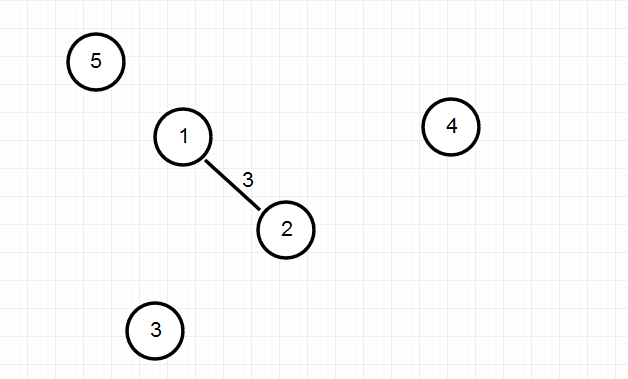第二次：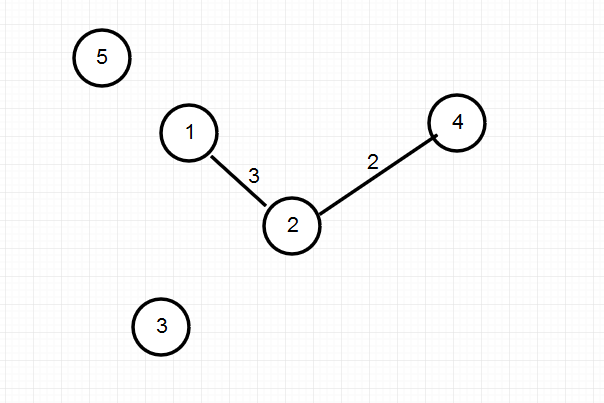第三次：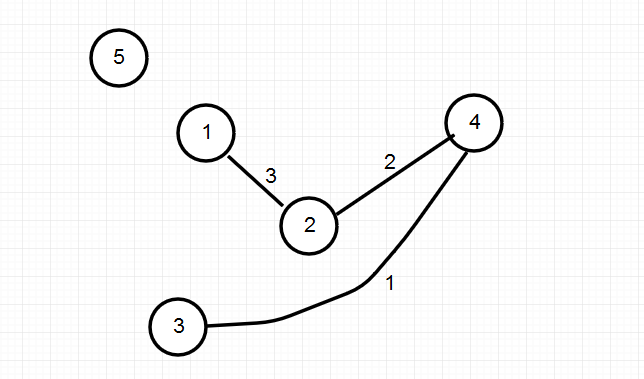第四次：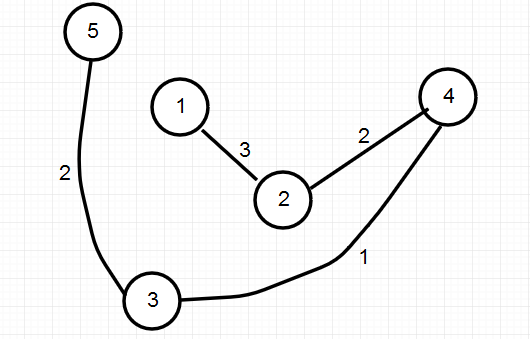一个最小生成树就构造好了。
$\bullet$例题（还是上面的例题）：
#include<stdio.h>
#include<string.h>
#include<algorithm>
#include<queue>
#include<vector>
#include<set>
#include<iostream>
#include<queue>
#include<string>
using namespace std;
const int maxn=1e3+10;
const int inf=999999999;
int n,m;
int mp[maxn][maxn];
int vis[maxn],dis[maxn];

int prim(int start)
{
int ans=0;
dis[start]=0;
for(int i=1;i<=m;i++){
int minn=inf;
int now=0;
for(int j=1;j<=m;j++){//在未加入树中的结点中找最小的边
if(!vis[j]&&dis[j]<minn){
minn=dis[j];
now=j;
}
}
ans+=dis[now];//将点和边加入树中
vis[now]=1;
for(int j=1;j<=m;j++){//更新与刚才找到的now点有边相连的点
if(!vis[j]&&mp[now][j]<dis[j])
dis[j]=mp[now][j];
}
}
return ans;
}

void init(){
for(int i=1;i<=m;i++){
dis[i]=inf;
for(int j=1;j<=m;j++){
mp[i][j]=inf;
}
mp[i][i]=0;
}
for(int i=1;i<=m;i++)vis[i]=0;
}

int main()
{
while(scanf("%d",&m)&&m){///m个点
init();
n=m*(m-1)/2;
for(int i=1;i<=n;i++){
int a,b,c;
scanf("%d%d%d",&a,&b,&c);
mp[a][b]=min(mp[a][b],c);
mp[b][a]=min(mp[a][b],c);
}
int ans=prim(1);
printf("%d\n",ans);
}
return 0;
}




展开全文• 算法的核心思想就是在图中所有的边权值出来TE，再找一端固定(自己设定)U={u…}的点集合，另一端v不在点集合中的权值最小的边，然后将该边并入集合TE中，并且将v并入U集合中，重复该步骤直到所有的点都在U中，就...数据结构
• 步骤 1、将给出的密钥去重与26个字母拼接成后，生成5X5的矩阵也称作密码表（矩阵中I和J位置相同） 2、将明文两个两个为一对儿 3、利用加密方法来将明文加密 加密详解 P1、P2同行： 对应的C1和C2分别是紧靠P1、P2右端...
• 本文通过深入分析Rijndael算法，改进了算法的几个有可能产生不安全隐患的步骤，首先是对于最可能被攻击的混（MixColumns）进行的优化，使该步骤变成简单的查表而不是域乘，增加了非线形安全性；而对于子密钥的生成...
• 其中x即为新生成的数据序列，a，u为待定系数，a代表的是发展系数，u代表灰作用量，这里将这两个参数称作“灰参数”，用一个向量表示 3、对累加生成的数据做均值生成B与常数项Y 4、根据最小二乘法求得灰参数 5...经验分享
• 参阅了之前11级和12级学长/学姐的博客，个人认为证明是错误的，所以自己整理了一个版本（分歧点在了算法正确性分析的后面）。因为算法正确性的证明实在太麻烦，所以部分步骤有省略，望海涵。 0. 习题：分析以下...
• 为了在同学们遇到不熟悉或比较困难的算法时提供帮助，我们逐个步骤地描述每一个算法。此外，为了便于大家理解书中对算法的分析，对于其中所需的数学知识，我们给出了详细的解释。如果对某一主题已经有所了解，会发现...
• 为了在同学们遇到不熟悉或比较困难的算法时提供帮助，我们逐个步骤地描述每一个算法。此外，为了便于大家理解书中对算法的分析，对于其中所需的数学知识，我们给出了详细的解释。如果对某一主题已经有所了解，会发现...
• 22、 对于上图给出的有向图，写出最小成本生成树，给出求解算法。 动态规划 23、 求出上图中每对结点间的最短距离的算法，并给出计算结果。 24、 下图中给出了一个地图，地图中每个顶点代表一个城市，两个城市间的...
• 一、游戏介绍： 「数独sudoku」来自日文，但... 二、数独算法主要步骤1、 出题（1）静态数组，数值固定，或采用由用户输入方式（2）电脑出题算法将一行或者一随机填上1-9，生成一个数独题目 根据第一步生成的数独题c# query winform
• 在非确定算法A的猜测阶段生成一个字符串S=C1C2.Cq在验证阶段把字符串S视为一个可能的着色方案即把S视为一个颜色序列把颜色Ci赋给顶点Vi(i=1.q)如果满足着色条件即相邻顶点不同色则返回true值算法的具体步骤为 1 检查...
• 回溯法：在递归构造中，生成和检查的过程可以有机结合起来，从而减少不必要的枚举。把问题分解为若干个步骤求解时，如果当前步骤没有合法选择，则函数将返回上一级的递归调用，该现象称为回溯法。所以递归枚举通常被...
• 3）AStar-EightDigital文件夹：输入源状态和目标状态，程序搜索出P(n)与W(n)分别作为启发函数时的生成节点数以及扩展节点数，并给出从源状态到目标状态的移动步骤；运行界面如下： 提高了运行速度的几处编码...
• 使用回溯算法完成数独的求解与生成。 GitHub 仓库 : 数独的规则 数独的形式是下面这样的，一共有 81 个格子。 解数独时需要根据 9×9 盘面上的已知数字，推理出所有剩余空格的数字，并满足每一行、每一、每一个...C++
• R中分层聚类算法：创建数据集library(stats)#cbind： 根据进行合并，即叠加所有，m的矩阵与n的矩阵cbind()最后变成m+n，合并前提：cbind(a, c)中矩阵a、c的行数必需相符#rbind： 根据行进行合并，就是行的...
•  算法：1：初始化一个点a，并把a加入候选点数组point[]中，将所有可能与a相连的点于数组array；2：在array中选边，array[]>的权值最小的边，array[i]>；3：在图中找权值比array中还小的边替代array中的边；3：...distance date ini 扩展
• 本周邀请同济大学教授、航空AI大赛冠军团队导师带来应用算法优化航空运营...从问题的定义，到目标、约束剖析，再到模型的具体形式，以及大规模线性规划问题求解的列生成方法。 直播主题：应用算法优化航空运营 直播...
• 通过引文对物体检测论文进行排名 上次更新时间：2019年6月18日 研究了用于对象检测（边界框，语义分割，实例分割）的算法列表（带有...*仅当您要运行对列表进行排序并生成图表的python脚本时，才需要执行以下步骤：）
• 在棋盘上放置8个皇后，使得它们互不攻击，此时每个皇后的攻击范围为同行同和同对角线，要求找出所有解。 递归函数将不再递归调用它自身，而是返回上一层调用，这种现象称为回溯（backtracking）。 当把问题分成...
• 算法步骤：随机生成n多数据，利用murmurhash，得到32位的hash值，通过2 de 10分桶，来计算package test; import java.util.HashMap; import java.util.Iterator; import java.util.Random; class jishuguzhashmap
• 更新计划：主要更新最近做项目课题时遇到的一些优化问题的源代码，以及一些步骤分析，涉及主要算法包括：分治法、动态规划、贪心法、回溯法、分支限界、列生成、分支定价、切平面法、分支定价切割… ...
• 管道有四个主要步骤： 基因型HLA 。 使用POLYSOLVER进行基因分型。 构建突变的肽。 对于非同义突变，基于HGVSc生成突变的肽序列。 注意：应该使用cmo_maf2maf --version 1.6.14 --vep-release 88使用此EXACT ...Python...